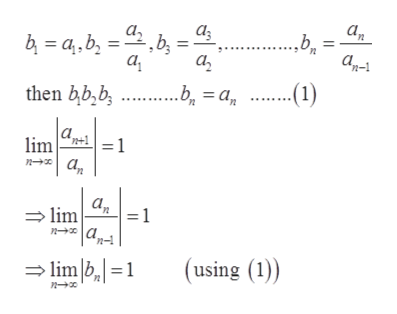# Write a proof for this theorem: Let the sum from n=1 to infinity of a-sub n be a series. Then the limit as n approaches infinity of the absolute value of a-sub (n+1) divided by a-sub n equals one if and only if the limit as n approaches infinity of the nth root of the absolute value of a-sub n equals one.

Question

Write a proof for this theorem:

Let the sum from n=1 to infinity of a-sub n be a series. Then the limit as n approaches infinity of the absolute value of a-sub (n+1) divided by a-sub n equals one if and only if the limit as n approaches infinity of the nth root of the absolute value of a-sub n equals one.

check_circleExpert Solution
Step 1

Given sum from n=1 to infinity of a-sub n be a series. Then

Step 2

Let use another sequence <bnhelp_outlineImage Transcriptioncloseа, а, а-1 а, а, (1) = a then bb,b .b а. lim n n+1 1 а, а, lim = 1 (using (1)) lim b,=1 fullscreen
Step 3

There exists a sequence <bn> such t...

### Want to see the full answer?

See Solution

#### Want to see this answer and more?

Solutions are written by subject experts who are available 24/7. Questions are typically answered within 1 hour*

See Solution
*Response times may vary by subject and question
Tagged in

### Other